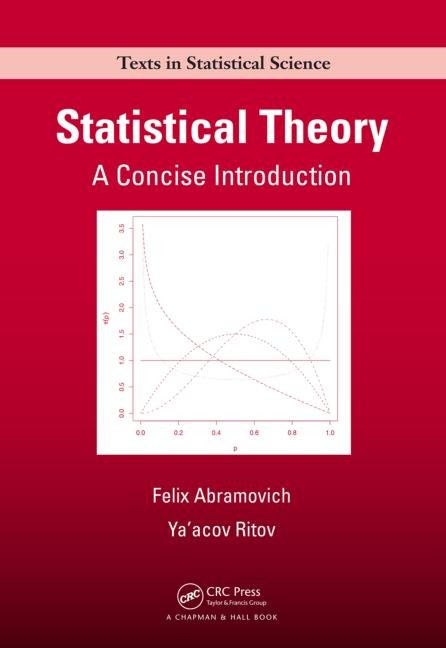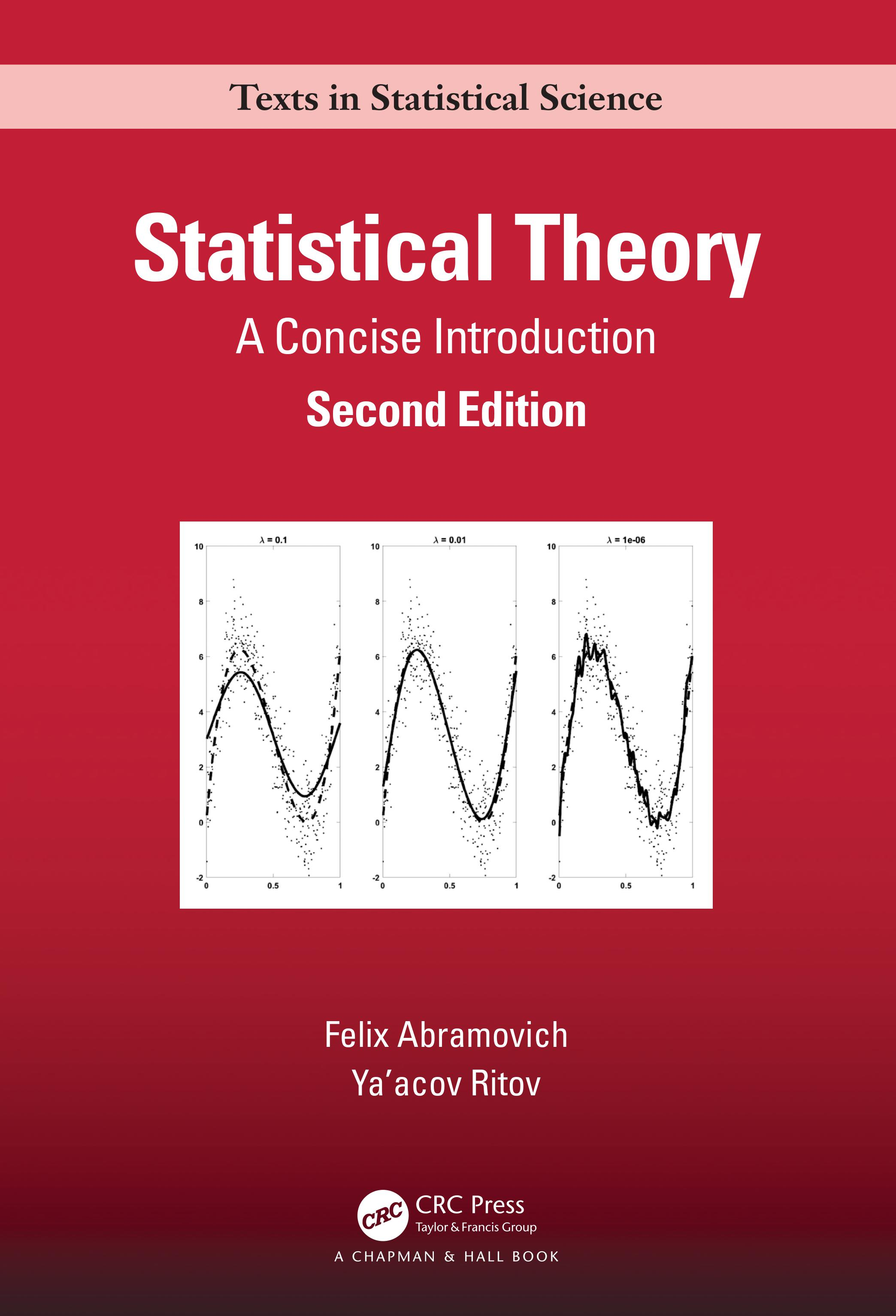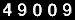### Felix Abramovich

#### Tel Aviv University

Ramat Aviv, Tel Aviv 69978
Israel
+972-3-6405389
Email: felix [at] tauex.tau.ac.il

#### Book##### Statistical Theory: A Concise Introduction (Second Edition)
Abramovich, F. and Ritov, Y. (2013, 2023).
Chapman & Hall/CRC
Summary: Designed for a one-semester advanced undergraduate or graduate course, Statistical Theory: A Concise Introduction clearly explains the underlying ideas and principles of major statistical concepts, including parameter estimation, confidence intervals, hypothesis testing, asymptotic analysis, Bayesian inference, linear models, nonparametric estimation, and elements of decision theory. It introduces these topics on a clear intuitive level using illustrative examples in addition to the formal definitions, theorems, and proofs. Based on the authors’ lecture notes, the book is self-contained, which maintains a proper balance between the clarity and rigor of exposition. In a few cases, the authors present a "sketched" version of a proof, explaining its main ideas rather than giving detailed technical mathematical and probabilistic arguments.

#### Research Interests:

• statistical learning
• high-dimensional inference, sparsity, model selection procedures
• nonparametric curve estimation and related problems
• wavelets in statistics

#### Professional Associations:

According to WEB counter you are Visitor No: